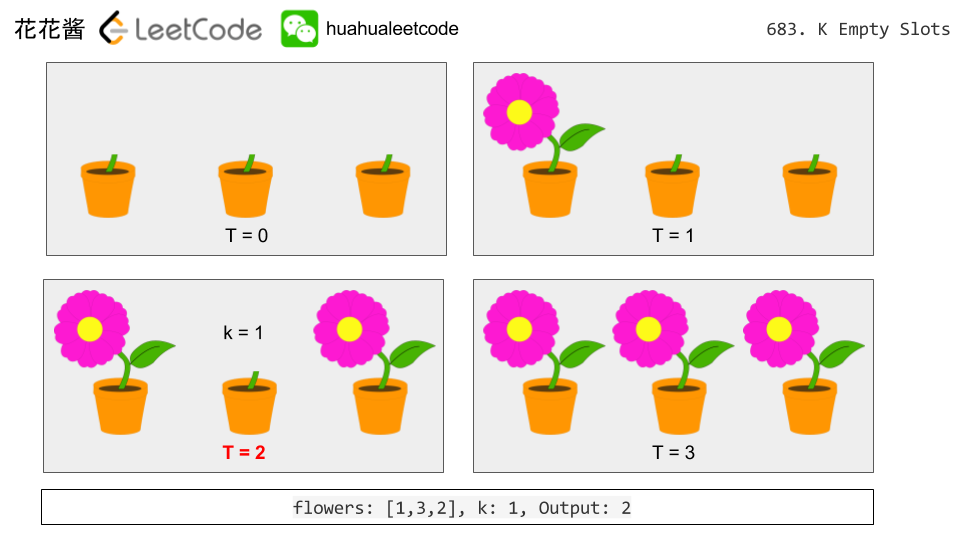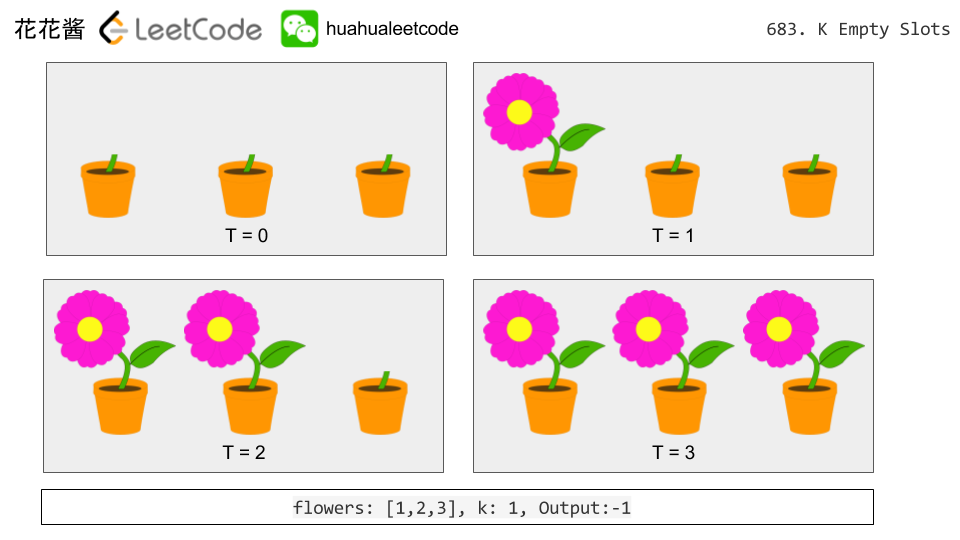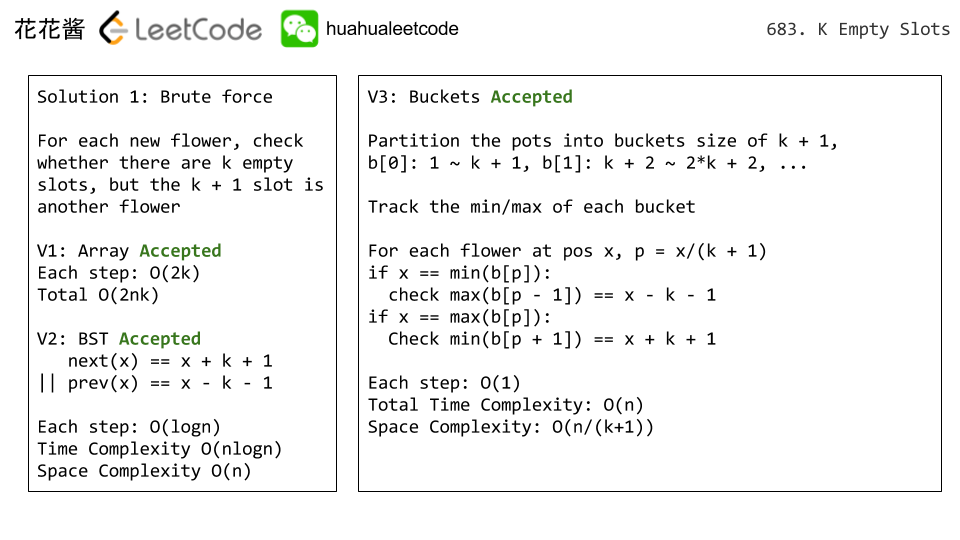Posts published in “Simulation”

Problem:

Initially, there is a Robot at position (0, 0). Given a sequence of its moves, judge if this robot makes a circle, which means it moves back to the original place.

The move sequence is represented by a string. And each move is represent by a character. The valid robot moves are R (Right), L (Left), U (Up) and D (down). The output should be true or false representing whether the robot makes a circle.

Example 1:

Example 2:

Idea:

Simulation

Solution:

C++

Problem:

There is a garden with N slots. In each slot, there is a flower. The N flowers will bloom one by one in N days. In each day, there will be exactly one flower blooming and it will be in the status of blooming since then.

Given an array flowers consists of number from 1 to N. Each number in the array represents the place where the flower will open in that day.

For example, flowers[i] = x means that the unique flower that blooms at day i will be at position x, where i and x will be in the range from 1 to N.

Also given an integer k, you need to output in which day there exists two flowers in the status of blooming, and also the number of flowers between them is k and these flowers are not blooming.

If there isn’t such day, output -1.

Example 1:

Input:
flowers: [1,3,2]
k: 1
Output: 2
Explanation: In the second day, the first and the third flower have become blooming.

Example 2:

Input:
flowers: [1,2,3]
k: 1
Output: -1

Note:

1. The given array will be in the range [1, 20000].

Idea:

BST/BucketsProblem:

https://leetcode.com/problems/next-closest-time/description/
no limit on how many times a digit can be reused.

You may assume the given input string is always valid. For example, “01:34”, “12:09” are all valid. “1:34”, “12:9” are all invalid.

Example 1:

Input: "19:34"
Output: "19:39"
Explanation: The next closest time choosing from digits 1, 9, 3, 4, is 19:39,
which occurs 5 minutes later.
It is not 19:33, because this occurs 23 hours and 59 minutes later.

Example 2:

Input: "23:59"
Output: "22:22"
Explanation: The next closest time choosing from digits 2, 3, 5, 9, is 22:22.
It may be assumed that the returned time is next day's time since it is smaller
than the input time numerically.

Related Problems:

Problem:

You’re now a baseball game point recorder.

Given a list of strings, each string can be one of the 4 following types:

1. Integer (one round’s score): Directly represents the number of points you get in this round.
2. "+" (one round’s score): Represents that the points you get in this round are the sum of the last two validround’s points.
3. "D" (one round’s score): Represents that the points you get in this round are the doubled data of the last valid round’s points.
4. "C" (an operation, which isn’t a round’s score): Represents the last valid round’s points you get were invalid and should be removed.

Each round’s operation is permanent and could have an impact on the round before and the round after.

You need to return the sum of the points you could get in all the rounds.

Example 1:

Example 2:

Note:

• The size of the input list will be between 1 and 1000.
• Every integer represented in the list will be between -30000 and 30000.

Idea:

Simulation

Solution:

C++:

Java:

Python

Mission News Theme by Compete Themes.